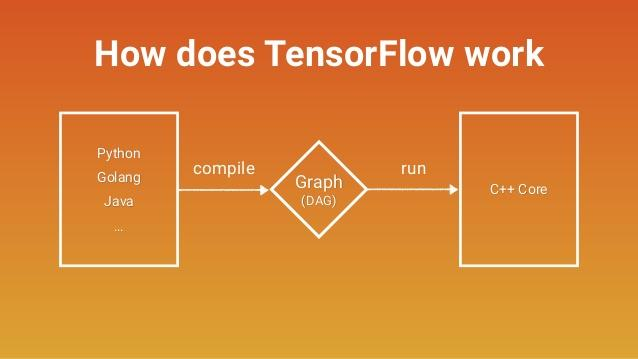## 第一个关键概念：计算图

``import tensorflow as tf``

``````import tensorflow as tf
two_node = tf.constant(2)
print two_node``````

``Tensor("Const:0", shape=(), dtype=int32)``

``````import tensorflow as tf
two_node = tf.constant(2)
another_two_node = tf.constant(2)
two_node = tf.constant(2)
tf.constant(3)
``````

``````import tensorflow as tf
two_node = tf.constant(2)
another_pointer_at_two_node = two_node
two_node = None
print two_node
print another_pointer_at_two_node``````

``````None
Tensor("Const:0", shape=(), dtype=int32)``````

``````import tensorflow as tf
two_node = tf.constant(2)
three_node = tf.constant(3)
sum_node = two_node + three_node ## 相当于tf.add(two_node, three_node)``````

## 第二个关键概念：会话

``````import tensorflow as tf
two_node = tf.constant(2)
three_node = tf.constant(3)
sum_node = two_node + three_node
sess = tf.Session()
print sess.run(sum_node)``````

``5``

``````import tensorflow as tf
two_node = tf.constant(2)
three_node = tf.constant(3)
sum_node = two_node + three_node
sess = tf.Session()
print sess.run([two_node, sum_node])``````

``[2, 5]``

``````import tensorflow as tf
input_placeholder = tf.placeholder(tf.int32)
sess = tf.Session()
print sess.run(input_placeholder)``````

``````Traceback (most recent call last):
...
InvalidArgumentError (see above for traceback): You must feed a value for placeholder tensor 'Placeholder' with dtype int32
[[Node: Placeholder = Placeholder[dtype=DT_INT32, shape=<unknown>, _device="/job:localhost/replica:0/task:0/device:CPU:0"]()]]
``````

``````import tensorflow as tf
input_placeholder = tf.placeholder(tf.int32)
sess = tf.Session()
print sess.run(input_placeholder, feed_dict={input_placeholder: 2})``````

``2``

## 第三个关键概念：计算路径

``````import tensorflow as tf
input_placeholder = tf.placeholder(tf.int32)
three_node = tf.constant(3)
sum_node = input_placeholder + three_node
sess = tf.Session()
print sess.run(three_node)
print sess.run(sum_node)
``````

``````3
Traceback (most recent call last):
...
InvalidArgumentError (see above for traceback): You must feed a value for placeholder tensor 'Placeholder_2' with dtype int32
[[Node: Placeholder_2 = Placeholder[dtype=DT_INT32, shape=<unknown>, _device="/job:localhost/replica:0/task:0/device:CPU:0"]()]]
``````

Tensorflow的框架优势离不开计算路径设计。想象一下，如果我们手里有一幅巨型计算图，其中包含大量不必要的节点，通过这样的计算方式，我们可以绕过大多数点，只计算必要内容，这就为节省大量运行时间提供了可能性。此外，它还允许我们构建大型的“多用途”计算图，这些图中可以有一些共享的核心节点，但我们可以通过不同计算路径来进行不同的计算。

``````import tensorflow as tf
count_variable = tf.get_variable("count", [])
sess = tf.Session()
print sess.run(count_variable)``````

``````Traceback (most recent call last):
...
tensorflow.python.framework.errors_impl.FailedPreconditionError: Attempting to use uninitialized value count
[[Node: _retval_count_0_0 = _Retval[T=DT_FLOAT, index=0, _device="/job:localhost/replica:0/task:0/device:CPU:0"](count)]]
``````

``````import tensorflow as tf
count_variable = tf.get_variable("count", [])
zero_node = tf.constant(0.)
assign_node = tf.assign(count_variable, zero_node)
sess = tf.Session()
sess.run(assign_node)
print sess.run(count_variable)``````

``0``

• 它不做计算，总是等于`value`
• 副作用（Side Effects）。上图显示了这个操作的副作用，当计算流通过`assign_node`时，`count_variable`节点里的值被强行替换成了`zero_node`节点的值；
• 即便`count_variable`节点和`assign_node`之间存在连接，但两者互不依赖（虚线）。

``````import tensorflow as tf
const_init_node = tf.constant_initializer(0.)
count_variable = tf.get_variable("count", [], initializer=const_init_node)
sess = tf.Session()
print sess.run([count_variable])``````

``````Traceback (most recent call last):
...
tensorflow.python.framework.errors_impl.FailedPreconditionError: Attempting to use uninitialized value count
[[Node: _retval_count_0_0 = _Retval[T=DT_FLOAT, index=0, _device="/job:localhost/replica:0/task:0/device:CPU:0"](count)]]
``````

``````import tensorflow as tf
const_init_node = tf.constant_initializer(0.)
count_variable = tf.get_variable("count", [], initializer=const_init_node)
init = tf.global_variables_initializer()
sess = tf.Session()
sess.run(init)
print sess.run(count_variable)``````

``0.``

## 优化

• 获得input和true_output
• 根据输入和参数计算一个估计值
• 对比true_output和估计值，计算模型loss
• 根据loss梯度更新参数

``````import tensorflow as tf

### 构建计算图
## 首先设置参数
m = tf.get_variable("m", [], initializer=tf.constant_initializer(0.))
b = tf.get_variable("b", [], initializer=tf.constant_initializer(0.))
init = tf.global_variables_initializer()

## 其次设置计算
input_placeholder = tf.placeholder(tf.float32)
output_placeholder = tf.placeholder(tf.float32)

x = input_placeholder
y = output_placeholder
y_guess = m * x + b

loss = tf.square(y - y_guess)

## 最后设置优化器和最小化节点
train_op = optimizer.minimize(loss)

### 启动会话
sess = tf.Session()
sess.run(init)

### 执行训练循环
import random

## 提出一个问题
true_m = random.random()
true_b = random.random()

for update_i in range(100000):
## (1) 获得输入和输出
input_data = random.random()
output_data = true_m * input_data + true_b

## (2), (3), (4) 一起调用一个sess.run()！
_loss, _ = sess.run([loss, train_op], feed_dict={input_placeholder: input_data, output_placeholder: output_data})
print update_i, _loss

### 最后，print计算得出的两个参数的更新值
print "True parameters:     m=%.4f, b=%.4f" % (true_m, true_b)
print "Learned parameters:  m=%.4f, b=%.4f" % tuple(sess.run([m, b]))``````

``````0 2.3205383
1 0.5792742
2 1.55254
3 1.5733259
4 0.6435648
5 2.4061265
6 1.0746256
7 2.1998715
8 1.6775116
9 1.6462423
10 2.441034
...
99990 2.9878322e-12
99991 5.158629e-11
99992 4.53646e-11
99993 9.422685e-12
99994 3.991829e-11
99995 1.134115e-11
99996 4.9467985e-11
99997 1.3219648e-11
99998 5.684342e-14
99999 3.007017e-11
True parameters:     m=0.3519, b=0.3242
Learned parameters:  m=0.3519, b=0.3242``````

``````## 最后设置优化器和最小化节点
train_op = optimizer.minimize(loss)``````

## 用`tf.Print`调试

``````import tensorflow as tf
two_node = tf.constant(2)
three_node = tf.constant(3)
sum_node = two_node + three_node
sess = tf.Session()
print sess.run(sum_node)``````

``5``

2加3是等于5，但如果我们想要检查的是`two_node``three_node`这两个值，又该怎么办？对此，一种可行的解决方法是在`sess.run()`中为每个要检查的节点添加返回参数，然后输出这些参数。

``````import tensorflow as tf
two_node = tf.constant(2)
three_node = tf.constant(3)
sum_node = two_node + three_node
sess = tf.Session()
answer, inspection = sess.run([sum_node, [two_node, three_node]])
print inspection

``````[2, 3]
5``````

``````import tensorflow as tf
two_node = tf.constant(2)
three_node = tf.constant(3)
sum_node = two_node + three_node
print_sum_node = tf.Print(sum_node, [two_node, three_node])
sess = tf.Session()
print sess.run(print_sum_node)``````

``````
5``````

``````import tensorflow as tf
two_node = tf.constant(2)
three_node = tf.constant(3)
sum_node = two_node + three_node
### 这个two_node的新副本不在计算路径上，所以最后没有print
print_two_node = tf.Print(two_node, [two_node, three_node, sum_node])
sess = tf.Session()
print sess.run(sum_node)``````

``5``